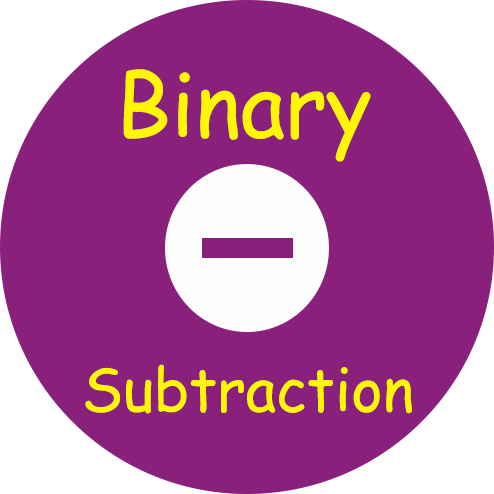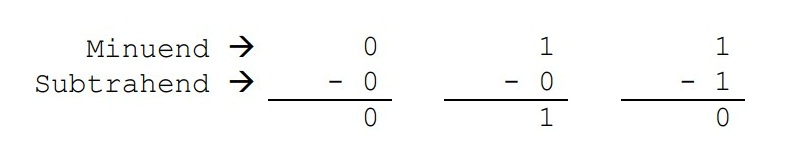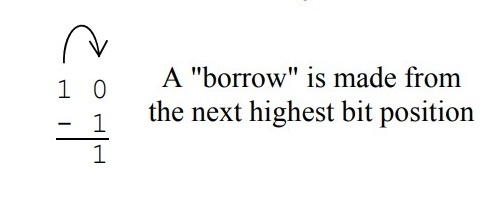﻿ Binary Subtraction Calculator | Subtract Two Binary Values

# Binary Subtraction Calculator

Use our Binary Subtraction Operation, a free online calculator / conversion tool to subtract two binary values. A Binary number system has only two digits that are 0 and 1. The base of binary number system is 2, because it has only two digits.

## Rules for Binary Subtraction

The rules for binary subtraction are
0 - 0 = 0
1 - 1 = 0
1 - 0 = 1
10 - 1 = 1 with a borrow of 1#### Binary Subtraction

Let's understand the process used in the binary numbering system for subtraction. There are four possible cases of single-bit binary subtraction:
0 – 0
0 – 1
1 – 0
1 – 1
As long as the value being subtracted from (the minuend) is greater than or equal to the value subtracted from it (the subtrahend), the process is contained in a single column.But what happens in the one case when the minuend is less than the subtrahend? As in decimal, a borrow must be taken from the next most significant digit. The same is true for binary.Pulling 1 from the next highest column in binary allows us to add 102 or a decimal 2 to the current column. For the previous example, 102 added to 0 gives us 102 or a decimal 2. When we subtract 1 from 2, the result is 1.

### Spreading Knowledge Across the World

USA - United States of America  Canada  United Kingdom  Australia  New Zealand  South America  Brazil  Portugal  Netherland  South Africa  Ethiopia  Zambia  Singapore  Malaysia  India  China  UAE - Saudi Arabia  Qatar  Oman  Kuwait  Bahrain  Dubai  Israil  England  Scotland  Norway  Ireland  Denmark  France  Spain  Poland  and  many more....